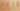RecursiveActivationsShareFrame

Misconception:

If a method is called recursively, a local variable of that method exists only once across the entire recursive computation. That is, the recursive invocations of a specific method all share the same activation record.Incorrect

Recursive calls of a method share a stack frame

Correct

Each recursive call of a method gets its own stack frame

CorrectionHere is what's right.

Each recursive call gets its own separate activation record/stack frame. Recursive calls are nested. Stack frames are allocated on method entry and freed on method return. Thus each recursive activation of a method has its own copies of the local variables and parameters defined in that method.

SymptomsHow do you know your students might have this misconception?

The following examples show possible symptoms of this misconception:

In the following example, maxValue is used to accumulate the maximum value stored in the nodes of a linked list (note that in this method the recursion has no base case):

int max() {
int maxValue = 0;
if (value>maxValue) {
maxValue=value;
}
return tail.max();
}

In the following example, total is an accumulator used across all recursive calls:

public int length() {
int total = 0;
if (this.tail==null) {
} else {
total = 1 + this.tail.length();
}
}

In the following method, the local variable c serves as an accumulator across the whole recursion.

int length() {
int c = 0;
if (tail!=null) {
c = c+1;
tail.length();
}
return c;
}

In the following example, the variable max is declared as static, possibly by a student who already knew C:

public int max() {
static int max = value;
if (tail==null) {
return max;
} else {
if (tail.value>max) {
max = tail.value;
}
return tail.max();
}
}

ValueHow can you build on this misconception?

This misconception isn’t so far-fetched. The idea of having a call stack to provide frames (local variable storage) for multiple activations of a given function was quite ingenious. Early programming languages (e.g., some implementations of FORTRAN’77 and earlier) statically allocated local variables (which meant that subroutines were not reentrant and recursion was not supported).

In his “Programming Loops vs Recursion” video, Computerphile’s Professor David Brailsford explains nicely how recursion helps with certain problems that nested loops (without the use of a stack) won’t be able to solve, the role of the call stack in recursion, and how early FORTRAN did not support recursion (at 7:40), because activations did share a stack frame:

Static local variables

In the C programming language, local variables can be declared static, which means that while their scope is the function in which they are declared, their lifetime extends to the duration of the program. Thus, in C a static local variable can be used to share information across recursive function calls.

Java does not have such variables: the lifetime of all local variables in Java is the lifetime of the activation record.

Instance or class variables

A recursive method might use the same instance or class variable across all the recursive invocations. An example of this is when using the Visitor pattern to accumulate some information. In such a design the Visitor may have an instance variable that holds the collected information.

Closures

This situation is not too different from using instance or class variables. A recursive function may be nested inside another function, and that outer function may have a local variable that is accessible and can be updated from within all invocations of the recursive method.

Note, though, that in Java local variables defined in enclosing scopes have to be effectively final to be usable in closures in Java.

Java